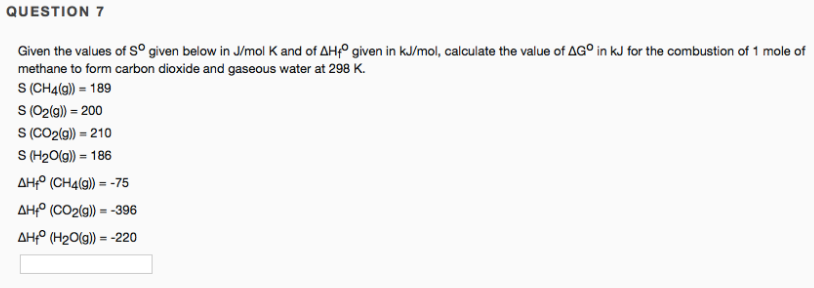# Given the values of S° given below in J/mol K and of ΔHf° given in kJ/mol, calculate the value of ΔG° in kJ for the combustion of 1 mole of methane to form carbon dioxide and gaseous water at 298 K. S (CH4(g)) = 189 S (O2(g)) = 200 S (CO2(g)) = 210 S (H2O(g)) = 186 ΔHf° (CH4(g)) = -75 ΔHf° (CO2(g)) = -396 ΔHf° (H2O(g)) = -220